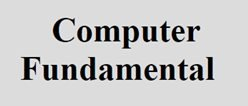# BCD Code, Excess-3 Code, 2421 Codes, Gray Code

Here we will talk about the four type of codes that is:

1. BCD code
2. Excess-3 code
3. 2421 code
4. Gray code

## Binary Coded Decimal (BCD) Code

Binary Coded Decimal (BCD) code is the simplest binary code to represent a decimal number.

In BCD code, a decimal number is represent by 4-binary bit. If a decimal number consist of two or more than two digit, then each decimal digit is individually represented by 4-bit binary equivalent. For example, (278)10 in BCD is represented as given in the following photo:Therefore we can say that the number 278 in binary is represented as 0010 0111 1000 or (278)10 = 0010 0111 1000.

BCD code is a weighed code, that is the weight of four binary bits which represent an individual digits are 8, 4, 2, 1 modern computer perform subtraction using complements and there is a difficulty in forming complements when number are represented by BCD codes.

## Excess-3 Code

In Excess-3 code, 3 is added to the individual digit of a decimal number then these binary equivalent are written. For example, (278)10 in excess-3 code is represented by 0101101011. This code is not a weighted code.

## 2421 Code

2421 code is another BCD code. It is weighed code. For example, 6 is 1100 and 3 is 0011.

## GRAY Code

The gray code is a binary code. The binary bits are arranged in such a way that only one binary bit changes at a time when we make a change from any number to the next.

Gray code is reflected code. This code is used in shaft encodes which indicates the angular position of a shaft in digital form. Shaft position encoder disks are used as sensors. It is not a weighted code. Gray code can be constructed using the following properties:

• A 1 bit gray code has two code words 0 and 1 to represent decimal number 0 and 1 respectively.
• A n bit gray code will have first 2n-1 gray codes for n-1 bits written in order with a leading 0 appended.
• The last 2n-1 gray code will be equal to the gray code words of an (n-1) bits gray code, written in reverse order (assuming a mirror placed between first 2n-1 and last 2n-1 gray codes) with a leading 1 appended.

## Example

The table given below shows an example of all the four type of code converted into each other.

Decimal No. BCD (8421) Excess-3 Code 2421 Code Gray Code
0 0000 0011 0000 0000
1 0001 0100 0001 0001
2 0010 0101 0010 0011
3 0011 0110 0011 0010
4 0100 0111 0100 0110
5 0101 1000 0101 0111
6 0110 1001 1100 0101
7 0111 1010 1101 0100
8 1000 1011 1110 1100
9 1001 1100 1111 1101
10 0001 0000 1111
11 0001 0001 1110
12 0001 0010 1010
13 0001 0011 1011
14 0001 0100 1001
15 0001 0101 1000

Tools
Calculator